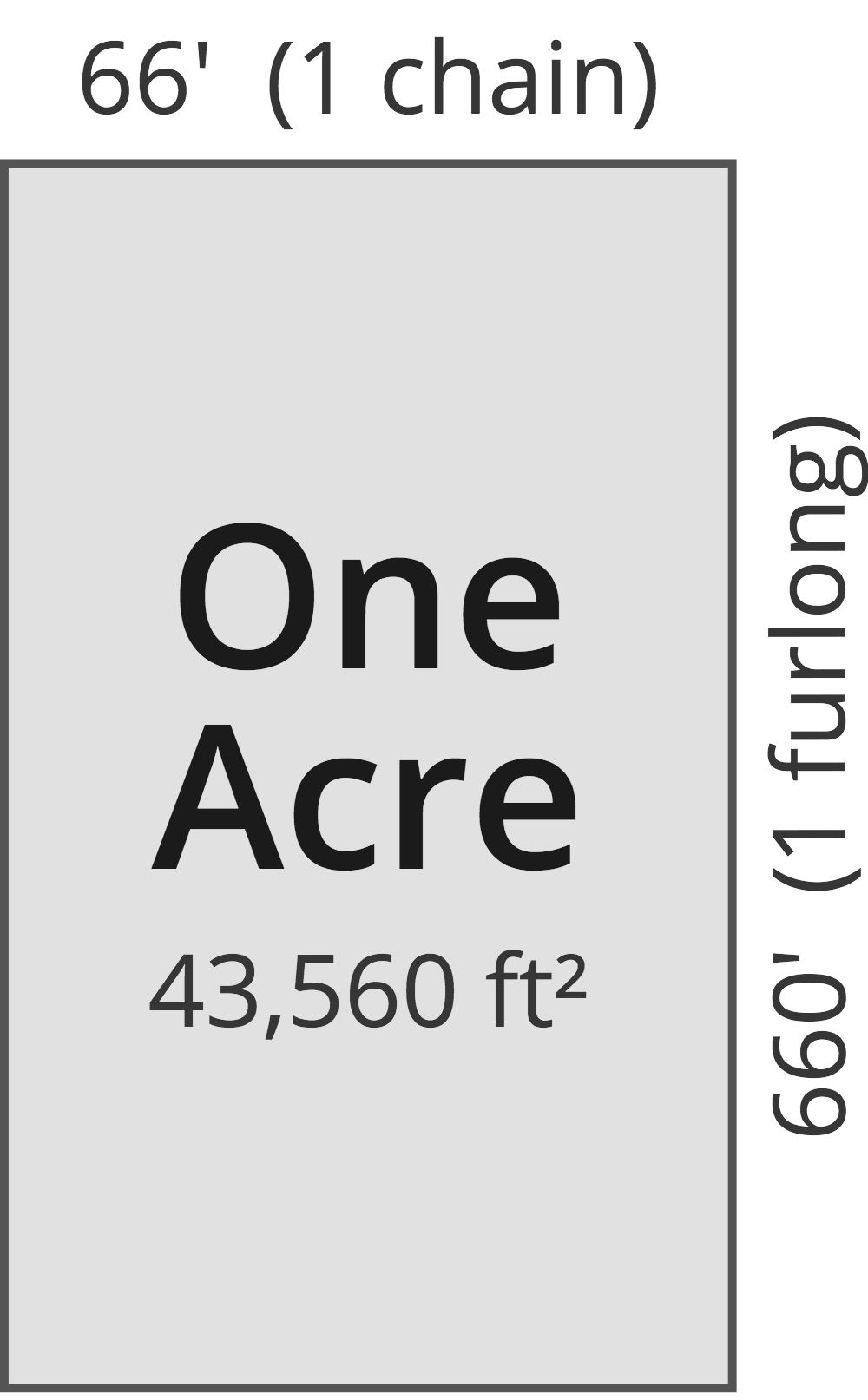# Acres to Square Inches Converter

Enter the area in acres below to get the value converted to square inches.

(find acres)
Results in Square Inches:1 ac = 6,272,640 sq in

Do you want to convert square inches to acres?

## How to Convert Acres to Square Inches

To convert a measurement in acres to a measurement in square inches, multiply the area by the following conversion ratio: 6,272,640 square inches/acre.

Since one acre is equal to 6,272,640 square inches, you can use this simple formula to convert:

square inches = acres × 6,272,640

The area in square inches is equal to the area in acres multiplied by 6,272,640.

For example, here's how to convert 5 acres to square inches using the formula above.
square inches = (5 ac × 6,272,640) = 31,363,200 sq in

### How Many Square Inches Are in an Acre?

There are 6,272,640 square inches in an acre, which is why we use this value in the formula above.

1 ac = 6,272,640 sq in

## What is an Acre?

One acre is defined as the area equal to a space that is one chain (66 ft) by one furlong (660 ft), or 10 square chains. That's equal to 43,560 square feet or 1/640 of a square mile for those unfamiliar with those units of measure.The acre is a US customary and imperial unit of area. Acres can be abbreviated as ac; for example, 1 acre can be written as 1 ac.

You can use an acreage calculator to measure the area of a plot of land in acres by locating the boundaries on a map.

## What is a Square Inch?

One square inch is equivalent to the area of a square with sides that are each 1 inch in length. One square inch is equal to 1/144 of a square foot or 1/1,296 of a square yard.

The square inch is a US customary and imperial unit of area. A square inch is sometimes also referred to as a square in. Square inches can be abbreviated as sq in, and are also sometimes abbreviated as in². For example, 1 square inch can be written as 1 sq in or 1 in².

## Acre to Square Inch Conversion Table

Table showing various acre measurements converted to square inches.
Acres Square Inches
0.000001 ac 6.2726 sq in
0.000002 ac 12.55 sq in
0.000003 ac 18.82 sq in
0.000004 ac 25.09 sq in
0.000005 ac 31.36 sq in
0.000006 ac 37.64 sq in
0.000007 ac 43.91 sq in
0.000008 ac 50.18 sq in
0.000009 ac 56.45 sq in
0.0000001 ac 0.627264 sq in
0.000001 ac 6.2726 sq in
0.00001 ac 62.73 sq in
0.0001 ac 627.26 sq in
0.001 ac 6,273 sq in
0.01 ac 62,726 sq in
0.1 ac 627,264 sq in
1 ac 6,272,640 sq in

## References

1. National Institute of Standards and Technology, Specifications, Tolerances, and Other Technical Requirements for Weighing and Measuring Devices, Handbook 44 - 2019 Edition, https://nvlpubs.nist.gov/nistpubs/hb/2019/NIST.HB.44-2019.pdf